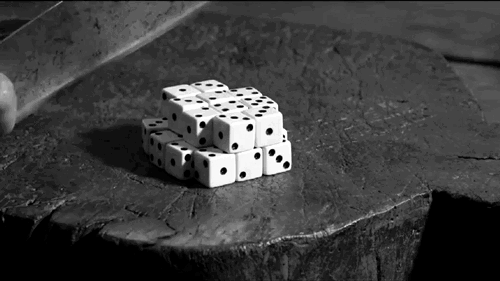# Dicey Probability with Dice!

Probability Level 3What is the probability that a roll of 5 distinct dice yields a sum of 17)? Round to 4 significant figures.

×